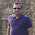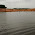## Saturday, 14 June 2014

### String Interview Questions and Answers

I have shared some String interview questions with answers so it can help for the job seekers.

1) Is String data type in Java?

No, String is a class, not data type.

2) How many ways to create a String object?

There are two ways to create the String object in java,

• String literal
• Using new Operator

3) What is difference between creating String as new and literal?

When we create string with new() Operator, it’s created in heap and not added into
string pool while String created using literal are created in String pool itself which exists
in PermGen area of heap.

4) What would be the output?

```  String s1="nrk";
String s2="nrk";
String s3=new String("nrk");

if(s1==s2){
System.out.println("s1 & s2 equal");
} else{
System.out.println("s1 & s2 not equal");
}

if(s2==s3){
System.out.println("s2 & s3 equal");
} else{
System.out.println("s2 & s3 not equal");
}```

Output:  s1 & s2 equal
s2 & s3 not equal

5) See below two variables what is difference?

```  String a="hcl technology";

final String a="hcl technology";
```

Here both are immutable but in first variable reassignment of variable  is  possible but in case of final it is not possible. It will give compile-time  error.

e.g          String a="hcl";
a=a.concat("b");            //no error,compile & run normally

in case of final,
final String a="hcl";
a=a.concat("b");             //give compile time error,reassignment not possible.

6)
```     System.out.println(2+3+"4");
System.out.println("2"+3+4);```

What would be the output for above two lines?

Output:--    54
234

7) What is the output of the given code?

``` public class A{
public static void main(String [] args) {
String s1 = null;
String s2 = s1+"java";
System.out.println(s2);
}
}
```

Output: nulljava

8) What is the output of the given code?

```    public class A {

public static void main(String [] args){

String s1 = null;

System.out.println(s1.length());

}

}
```

Output:--java.lang.NullPointerException

9) What is the output of the given code?

```    public class A {

public static void main(String [] args){

String s1 = null;

System.out.println(s1);

}

}
```
Output:--null

10) What is the output of the given code?

```    public class A {

public static void main(String [] args){

System.out.println(null);

}

}
```
Output:--Compile Time Error.

11) Write a program to swap two String variables without using the third variable .

```  public class StringSwap {

public static void main(String[] args) {

String a="var1";

String b="var2";

a= a+b;

b = a.substring(0,(a.length()-b.length()));

a = a.substring(b.length(),(a.length()));

System.out.println("a = "+a+" b="+b);   //a=var2 b=var1

}

}
```

12) Write a program to replace particular character with another character  from the given string without using Java API's.

``` public class ReplaceCh {

public static void main(String[] args) {

String a="Software";

String[] a1=a.split("");

String ch="";

for(int i=0;i<a1.length;i++){

if(a1[i].equals("w")){

ch=ch+"g";

} else{

ch=ch+a1[i];

}

}
System.out.println(ch);
}
}
```

it will print Softgare.

13) What would be the output?
```  public class StrEx {

public static void main(String[] args) {

StrEx g=new StrEx();

g.m(null);

}

public void m(String a){

System.out.println(a);

}

}
```
Output:-- null.

```public class StrEx {

public static void main(String[] args) {
m(null);
}

public static void m(String a){
System.out.println("String");
}

public static void m(int a){
System.out.println("int");
}

public static void m(Object o) {
System.out.println("Object");
}

}
```
What would be the output?

Output:- String.

```public class StrEx {

public static void main(String[] args) {
m(null);
}

public static void m(String a){
System.out.println("String");
}

public static void m(int a){
System.out.println("int");
}

public static void m(Object o) {
System.out.println("Object");
}

public static void m(Integer i){
System.out.println("Integer");
}

}
```
What would be the output?

Output:--Compile-time error at m(null)

16) Write a method  int Summation(String s). It will return summation of  integers from  the   given string.
e.g  String s1="12 3 4 some text 3";
output should be summation of 12,3,4 & 3=22.

```public int Summation(String s1) {
String[] s1 = a.split(" ");
int sum = 0;
for(int i = 0; i<s1.length; i++) {
if(s1[i].matches("[0-9]+")) {
sum = sum + Integer.parseInt(s1[i]);
}
}
return sum;
}
```

17) Write a program to find the length of the String without using the String method length.

``` public class Slength {

public int length(String s) {
int i=0;
try {
for(i=0;i>=0;i++) {
s.charAt(i);
}
}
catch(Exception e){
System.out.println("The length of the string ---");
}
return i;
}

public static void main(String[] args {
Slength h = new Slength();
int len = h.length("ghhjnnm");
System.out.println(len);
}
}
```

18) Write a program to count the letters from the given String.

``` public class CountLetter {

public static void countLetter(String s) {
if(s==null) {
return;
}
int counter=0;
for(int i=0;i<s.length();i++) {
if (Character.isLetter(s.charAt(i))) {
counter++;
}
}
System.out.println(counter);
}

public static void main(String[] args) {
CountLetter.countLetter("ggg999");
}
}
```

19) Write a program to check the given string is palindrome or not.

```import java.util.Scanner;
public class palindrome {```
`       public static void main(String[] args) {`
```
Scanner sc=new Scanner(System.in);
System.out.println("Enter a string");
String str=sc.nextLine();
int l=str.length();
String reverse="";
for(int i=l-1;i>=0;i--) {
reverse = reverse + str.charAt(i);
}
if(str.equals(reverse)) {
System.out.println("Palindrome");
}
else{
System.out.println("Not palindrome");
}
}
}
```

20) What is output?

``` public class test {
public static void main(String [] args) {
int x = 3;
int y = 1;
if (x = y) {
System.out.println("Not equal");
} else {
System.out.println("Equal");
}
}
}
```

Output:- Compile Time Error at if (x = y).

21) Does String is thread safe in java?

Strings are immutable, so we can’t change it’s value in program.  Hence it’s thread-safe and can be safely used in multi-threaded environment.

22)  Can we use String in switch case?

This is a tricky question used to check your knowledge of current Java developments. Java 7 extended the capability of switch case to use Strings also, earlier java versions doesn’t support this.
If you are implementing conditional flow for Strings, you can use if-else conditions and you can use switch case if you are using Java 7 or higher versions.

23) Why String is immutable or final in Java?

Related Posts:--
1) What are the immutable classes in Java? How to create immutable class and What are the conditions?
2) Why String is immutable or final in java?

1.Excellent
Clear some miss understanding

2.Thanks for comment...If u have any questions or doubt please share it in the blog..

3.Very neat and clearly presented. Thanks for sharing.

4.perfect question and answers very use ful thank you very much

5.thanks for your comment. If you have any doubt please comment in the blog post or send email to anilnivargi49@gmail.com

6.Thank you very much for sharing your knowledge.It is usefully for me.

1.